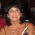## Monday, July 23, 2012

### Matrix Multiplication

Another quick tip. This time how to multiply matrices.

Begin with the matrix multiplication problem:

Then move the first matrix down. [Note: Since matrix multiplication is not commutative, this is important. Although it should be noted that the same effect can be accomplished by moving the second matrix up. But under no circumstances should the reverse be tried.] The answer will go in the new space you have created in the bottom right corner. Immediately you can see (if the product is possible) the shape of the answer. In this example it is a 2x2 matrix.

Pick a position in the answer matrix and follow across from the left and vertically from above to figure out which numbers you will use. Multiply pairs beginning with the outermost numbers (the blue 1 and 7 in the example) and sum with the product of the next pair in until you run out of pairs. The answer will go in the position where the arrows meet.

Remember not to use numbers from your answer when computing other spaces. For example, the 58 was not used to find the 64 below.

Continue with each position until the answer matrix is complete!

But what if the matrices in question are not able to be multiplied?

Consider the following case. Although it initially looks like our answer will be a 2x2 matrix, we see that the 3 does not have a pair, so these matrices cannot be multiplied in this order.

#### 3 comments:

1.Lovely method - thanks!

2.I am so glad that I saw this- seriously why doesn't everyone teach it this way. My students will be glad you posted this!

3.Not sure why this is considered so new. I have always done this.....